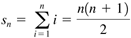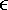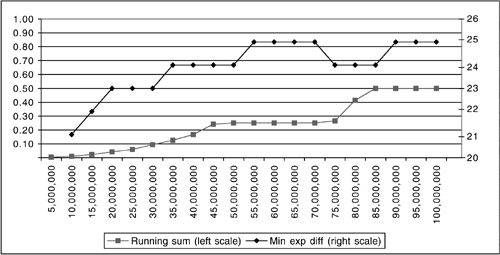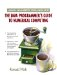# 4.1 A Summing Mystery?athe Magnitude ProblemJava Number Cruncher: The Java Programmer's Guide to Numerical Computing By Ronald  Mak Table of Contents Chapter  4.   Summing Lists of Numbers

4.1 A Summing Mystery?athe Magnitude Problem

Let's start with a simple summing problem. The formula for the sum s n of the first n counting numbers, 1 through n, isFor example, the sum of the first 10 counting numbers is 55.

Program 4-1 adds a slight twist to the summation. See Listing 4-1. It adds fractions instead, where, for each summation, the numerators are the first n counting numbers and the denominator is the sum s n . Therefore, the sum should be 1. For example, the fraction sum for s 10 isThe program computes the fraction sums with denominators s 10 , s 100 , s 1000 , and so on through s 100,000,000 .

We can see from the output that things go fairly smoothly, with the computed sums close to 1, until denominator s 100,000,000 ?aand disaster strikes. In the last line of output, how did the sum manage to be 0.5? That's not even close!

##### Listing 4-1 Fraction sums.
` package numbercruncher.program4_1; import numbercruncher.mathutils.AlignRight; /**  * PROGRAM 4-1: Fraction Sums  *  * For each n, compute the sum 1/d + 2/d + 3/d + ... + n/d = d/d  * where:  *     n = 10, 100, 1000, ..., 100,000,000  *     d = 1 + 2 + 3 + ... + n = (n/2)(n + 1)  *  * See how close each sum is to 1.  */ public class FractionSums {     public static void main(String args[])     {         AlignRight ar = new AlignRight();         ar.print("n", 9); ar.print("Denom", 14);         ar.print("1/Denom", 14); ar.print("n/Denom", 13);         ar.print("Sum", 11); ar.print("% Error", 13);         ar.underline();         for (int n = 10; n <= 100000000; n *= 10) {             float sum   = 0;             float denom = (0.5f*n)*(n + 1);             // Sum fractions.             for (int i = 1; i <= n; ++i) sum += i/denom;             ar.print(n, 9); ar.print(denom, 14); ar.print(1/denom, 14);             ar.print(n/denom, 13); ar.print(sum, 11);             ar.print(100*Math.abs(sum - 1), 13);             ar.println();         }     } } `

Output:

` n         Denom       1/Denom      n/Denom        Sum      % Error ---------------------------------------------------------------------------        10          55.0   0.018181818   0.18181819 0.99999994 5.9604645E-6       100        5050.0   1.980198E-4   0.01980198        1.0          0.0      1000      500500.0   1.998002E-6  0.001998002 0.99999994 5.9604645E-6     10000      5.0005E7     1.9998E-8    1.9998E-4  0.9999999 1.1920929E-5    100000   5.0000502E9 1.9999799E-10   1.99998E-5 0.99999875 1.2516975E-4   1000000 5.00000489E11  1.999998E-12  1.999998E-6  0.9998997  0.010031462  10000000  5.0000004E13 1.9999998E-14 1.9999999E-7   1.002663   0.26630163 100000000  5.0000001E15 1.9999999E-16       2.0E-8        0.5         50.0 `

Why should the fraction sum for denominator s 100,000,000 fail so miserably when the previous sums all did so well? It isn't underflow or overflow. For each sum, the program prints out the values of the first fraction and last fraction, and their exponent values are well within the range (-45 through +38) for the float type. Each sum is computed from many addends, and so we might expect most of the high and low roundoff errors to cancel each other out.

For one possible source of the problem, recall our computation of the machine epsilonat the end of Chapter 3. Since the sum is supposed to be 1, and the floatvalue is around 6.0 x 10 - 8 , we can see that, with denominator s 100,000,000 , a significant percentage of the addends may have no effect on the sum as it approaches 1.

Program 4-2 provides some insight. See Listing 4-2. It computes the fraction sum for denominator s 100,000,000 by dividing the series of fractions into 20 equal- sized groups, and at the end of each group it prints the current fraction and the current value of the running sum. Before adding each fraction to the running sum, it calculates the difference between the fraction's binary exponent and the running sum's binary exponent. At the end of each group, it also prints the minimum exponent difference of that group and the percentage of exponent differences within the group that exceeded 24. (Recall that, at the end of Chapter 3, we saw that, for type float, the unbiased binary exponent of the machine epsilon is -24.)

##### Listing 4-2 Fraction sums for n = 100,000,000.
` package numbercruncher.program4_2; import numbercruncher.mathutils.AlignRight; /**  * PROGRAM 4-2: Fraction Sum 100M  *  * Compute the sum 1/d + 2/d + 3/d + ... + n/d = d/d  * where:  *     n = 100,000,000  *     d = 1 + 2 + 3 + ... + n = (n/2)(n + 1)  *  * See why the sum ends up being 0.5  */ public class FractionSum100M {     private static final int GROUPS = 20;     private static final int MAX    = 100000000;    // 100M     public static void main(String args[])     {         AlignRight ar = new AlignRight();         ar.print("i", 9);         ar.print("Fraction", 15);         ar.print("Running sum", 15);         ar.print("Min exp diff", 15);         ar.print("% ExpDiff>24", 15);         ar.underline();         float sum   = 0;         float denom = (0.5f*MAX)*(MAX + 1);         int gSize   = MAX/GROUPS;         // group size         int gEnd    = gSize;              // index of group end         int minDiff = Integer.MAX_VALUE;  // min exponent difference         int exceeds = 0;                  // # of exponent diff > 24         // Loop to sum the fractions.         for (int i = 1; i <= MAX; ++i) {             float fraction = i/denom;             // Compute the exponent difference.             int expSum      = Float.floatToIntBits(sum)      >> 23;             int expFraction = Float.floatToIntBits(fraction) >> 23;             int diff        = Math.abs(expSum - expFraction);             if (i > 1) {                 minDiff = Math.min(minDiff, diff);                 if (diff > 24) ++exceeds;             }             sum += fraction;             // Printout at the end of each group.             if (i == gEnd) {                 ar.print(i, 9);                 ar.print(fraction, 15);                 ar.print(sum, 15);                 ar.print(minDiff, 15);                 ar.print((100*exceeds)/gSize, 15);                 ar.println();                 minDiff = Integer.MAX_VALUE;                 exceeds = 0;                 gEnd += gSize;             }         }     } } `

Output:

` i       Fraction    Running sum   Min exp diff   % ExpDiff>24 ---------------------------------------------------------------------   5000000         1.0E-9    0.002493547              0              0  10000000         2.0E-9    0.009991142             21              0  15000000         3.0E-9    0.022081949             22              0  20000000         4.0E-9      0.0407084             23              0  25000000         5.0E-9     0.05933485             23              0  30000000         6.0E-9     0.09342261             23              0  35000000   6.9999997E-9          0.125             24             15  40000000         8.0E-9     0.16593489             24             45  45000000         9.0E-9      0.2404407             24              0  50000000         1.0E-8           0.25             24             87  55000000         1.1E-8           0.25             25            100  60000000         1.2E-8           0.25             25            100  65000000   1.2999999E-8           0.25             25            100  70000000   1.3999999E-8           0.25             25            100  75000000         1.5E-8     0.26472795             24             90  80000000         1.6E-8     0.41373956             24              0  85000000         1.7E-8            0.5             24             42  90000000         1.8E-8            0.5             25            100  95000000         1.9E-8            0.5             25            100 100000000         2.0E-8            0.5             25            100 `

Now we begin to see what happened . If the difference in magnitude between two addends is too great, the smaller addend loses much of its contribution to the sum. Indeed, as we saw at the end of Chapter 3, smaller addends will have no effect at all on the sum if the difference between their binary exponents is greater than 24 when doing float addition.

Program 4-2 shows that, whenever the value of the running sum reaches the next power of 2, the sum's binary exponent increases by 1. If that widens the exponent difference between the sum and the upcoming fractions to more than 24, then those fractions have no effect on the sum until they become large enough to lower the exponent difference. Thus, the running sum "stalls" at 0.25 and again at 0.5 when the minimum exponent differences are 25. Figure 4-1 shows this clearly by graphing the values of the running sum versus the minimum exponent differences in each group.

##### Figure 4-1. A graph of the output of Program 4 §C2. The running sum "stalls" at 0.25 and at 0.5 when the minimum exponent differences exceed 24.Would it make a difference if we summed the fractions in the reverse order, from the largest down to the smallest? Program 4-3 shows that the running sum still stalls at 0.5, and we have even greater percentages of exponent differences exceeding 24. See Listing 4-3. So we've made the problem worse ?athe values of the running sum increase while the fractions decrease, making the exponent differences even larger.

In general, when adding a sorted list of numbers all of the same sign, it is better to add them in the order from the smallest magnitude up to the largest.

##### Listing 4-3 Fraction sums for n = 100,000,000 in reversed order.
` package numbercruncher.program4_3; import numbercruncher.mathutils.AlignRight; /**  * PROGRAM 4-3: Fraction Sum 100M in the Reversed Order  *  * Compute the sum n/d + (n-1)/d + (n-2)/d + ... + 2/d + 1/d = d/d  * where:  *     n = 100,000,000  *     d = 1 + 2 + 3 + ... + n = (n/2)(n + 1)  *  * See if the sum is closer to 1.  */ public class FractionSum100MReversed {     private static final int GROUPS = 20;     private static final int MAX    = 100000000;    // 100M     public static void main(String args[])     {         AlignRight ar = new AlignRight();         ar.print("i", 9);         ar.print("Fraction", 15);         ar.print("Running sum", 15);         ar.print("Min exp diff", 15);         ar.print("% ExpDiff>24", 15);         ar.underline();         float sum   = 0;         float denom = (0.5f*MAX)*(MAX + 1);         int gSize   = MAX/GROUPS;         // group size         int gStart  = (MAX + 1) - gSize;  // index of group start         int minDiff = Integer.MAX_VALUE;  // min exponent difference         int exceeds = 0;                  // # of exponent diff > 24         // Loop to sum the fractions.         for (int i = MAX; i >= 1; - i) {             float fraction = i/denom;             int expSum      = Float.floatToIntBits(sum)      >> 23;             int expFraction = Float.floatToIntBits(fraction) >> 23;             int diff        = Math.abs(expSum - expFraction);             if (i < MAX) {                 minDiff = Math.min(minDiff, diff);                 if (diff > 24) ++exceeds;             }             sum += fraction;             // Printout at the start of each group.             if (i == gStart) {                 ar.print(i, 9);                 ar.print(fraction, 15);                 ar.print(sum, 15);                 ar.print(minDiff, 15);                 ar.print((100*exceeds)/gSize, 15);                 ar.println();                 minDiff = Integer.MAX_VALUE;                 exceeds = 0;                 gStart -= gSize;             }         }     } } `

Output:

` i       Fraction    Running sum   Min exp diff   % ExpDiff>24 ---------------------------------------------------------------------  95000001         1.9E-8     0.10206167              0              0  90000001         1.8E-8     0.18421358             22              0  85000001         1.7E-8     0.26743877             23              0  80000001         1.6E-8     0.41645038             24              0  75000001         1.5E-8            0.5             24             43  70000001   1.3999999E-8            0.5             25            100  65000001   1.2999999E-8            0.5             26            100  60000001         1.2E-8            0.5             26            100  55000001         1.1E-8            0.5             26            100  50000001         1.0E-8            0.5             26            100  45000001         9.0E-9            0.5             26            100  40000001         8.0E-9            0.5             26            100  35000001   6.9999997E-9            0.5             26            100  30000001         6.0E-9            0.5             27            100  25000001         5.0E-9            0.5             27            100  20000001         4.0E-9            0.5             27            100  15000001         3.0E-9            0.5             27            100  10000001   2.0000002E-9            0.5             28            100   5000001   1.0000002E-9            0.5             28            100         1  1.9999999E-16            0.5             29            100 `

We have a clear challenge. In order for a summation to be accurate, we need to keep the running sum and each addend sufficiently close together in magnitude, so that the difference between their binary exponents is not greater than 24.

Program 4-4 shows one way to do this. See Listing 4-4. The program partitions the fractions into 20 equal-sized groups. It computes a separate subtotal for each group; then it adds the subtotal to the running sum. Each group's fractions are closer in magnitude to the group subtotal, so the minimum difference between the binary exponents of a fraction and its group subtotal is under 24. Each group subtotal has enough "heft" to make its contribution to the overall sum. The trick is to get the right size for the groups. The final sum is very close to 1.

##### Listing 4-4 Fraction sums for n = 100,000,000 using group subtotals.
` package numbercruncher.program4_4; import numbercruncher.mathutils.AlignRight; /**  * PROGRAM 4-4: Fraction Sum 100M Using Group Subtotals  *  * Compute the sum 1/d + 2/d + 3/d + ... + n/d = d/d  * where:  *     n = 100,000,000  *     d = 1 + 2 + 3 + ... + n = (n/2)(n + 1)  *  * Compute the sum by adding the group subtotals.  * See if the final sum is closer to 1.  */ public class FractionSum100MGrouped {     private static final int GROUPS = 20;     private static final int MAX    = 100000000;    // 100M     public static void main(String args[])     {         AlignRight ar = new AlignRight();         ar.print("i", 9);         ar.print("Group sum", 15);         ar.print("Running sum", 15);         ar.print("% ExpDiff>24", 15);         ar.underline();         float sum      = 0;           // running sum         float subtotal = 0;           // group subtotal         int   gSize    = MAX/GROUPS;  // group size         int   gEnd     = gSize;       // index of group end         int   exceeds  = 0;           // # of exponent diff > 24         float denom = (0.5f*MAX)*(MAX + 1);         // Sum the fractions by groups.         for (int i = 1; i <= MAX; ++i) {             float fraction = i/denom;             int expSubtotal = Float.floatToIntBits(subtotal) >> 23;             int expFraction = Float.floatToIntBits(fraction) >> 23;             int diff        = Math.abs(expSubtotal - expFraction);             if ((subtotal > 0) && (diff > 24)) ++exceeds;             subtotal += fraction;             // Subtotal and printout at the end of each group.             if (i == gEnd) {                 sum += subtotal;                 ar.print(i, 9);                 ar.print(subtotal, 15);                 ar.print(sum, 15);                 ar.print((100*exceeds)/gSize, 15);                 ar.println();                 subtotal = 0;                 exceeds  = 0;                 gEnd += gSize;             }         }         System.out.println("\n% error = " + 100*Math.abs(sum - 1));     } } `

Output:

` i      Group sum    Running sum   % ExpDiff>24 ------------------------------------------------------   5000000    0.002493547    0.002493547              0  10000000    0.007556428   0.0100499755              0  15000000    0.012413543     0.02246352              0  20000000    0.017349107    0.039812624              0  25000000    0.023679595     0.06349222              0  30000000     0.02718998      0.0906822              0  35000000    0.034128636     0.12481084              0  40000000    0.036770158       0.161581              0  45000000     0.04185812     0.20343912              0  50000000    0.049500026     0.25293913              0  55000000     0.05435951     0.30729866              0  60000000    0.055825584     0.36312425              0  65000000     0.05929653     0.42242077              0  70000000     0.07003953      0.4924603              0  75000000     0.07346739      0.5659277              0  80000000     0.07455075     0.64047843              0  85000000     0.07784402     0.71832246              0  90000000     0.08436947     0.80269194              0  95000000    0.098553196      0.9012451              0 100000000     0.09986824      1.0011134              0 % error = 0.11134148 `TopJava Number Cruncher: The Java Programmers Guide to Numerical Computing
ISBN: 0130460419
EAN: 2147483647
Year: 2001
Pages: 141
Authors: Ronald Mak

Similar book on Amazon